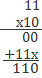## Binary Arithmetic and Conversion

Subject: Computer

#### Overview

You have to learn addition, subtraction, multiplication, and division of binary number. In brain, you have to keep that in the arithmetic of binary number, carry is written in binary (2) just like as 10 is used in decimal system for carry. This note provides an information about binary arithmetic.

#### Conversion from Decimal to Binary

To convert the decimal number to binary number, repeated division by two is needed.

Examples: Convert following into binary numbers:

1. (45)10= (101101)2
 2 45 1 2 22 0 2 11 1 2 5 1 2 2 0 1

=(101101)2

2. (225)10= (10111001)2
 2 225 1 2 112 0 2 56 0 2 23 1 2 11 1 2 5 1 2 2 0 1

= (10111001)2#### Conversion from Binary to Decimal

To convert binary to the decimal number system, multiply given number by 2.

1. (1101)2=(?)10

=1x23+ 1 x 22+ 0x 21+ 1x 20
=8 + 4 + 0 + 1
=13

 A B A+B 0 0 0 0 1 1 1 0 1 1 1 0 with carry 1
Examples:
1. 1100 + 1111= 11011
 1 Carry 1 1 0 0 1 1 1 1 11 0 1 1

#### Rules for Binary Multiplication

 A B A*B 0 0 0 0 1 0 1 0 0 1 1 1
Example:##### Things to remember
• To convert decimal number to binary number, repeated division by two is needed.
• To convert binary to decimal number system, multiply given number by 2.
• It includes every relationship which established among the people.
• There can be more than one community in a society. Community smaller than society.
• It is a network of social relationships which cannot see or touched.
• common interests and common objectives are not necessary for society.
##### Videos for Binary Arithmetic and Conversion

Binary numbers are added to the same manner as decimal numbers. There are only four possible combinations resulting from the addition of two binary digits.

 A B A+B 0 0 0 0 1 1 1 0 1 1 1 10

i.e. 0 carry 1

The rules for subtraction are the same in the binary system as in the decimal system. The result of the subtraction of binary digit is either 0 or 1.

Rules for binary subtraction:

 A B A-B 0 0 0 1 0 1 1 1 0 0 1 1
i.e. due to borrowing from the next column

Binary numbers are multiplied in the same manner as decimal numbers. We need to remember the rules of binary addition.

Rules for binary multiplication:

 A B A×B 0 0 0 1 0 0 0 1 0 1 1 1

=1x23+ 1 x 22+ 1x 21+ 0x 20
=8 + 4 + 2 + 0
=14

 2 45 1 2 22 0 2 11 1 2 5 1 2 2 0 1

=(101101)2